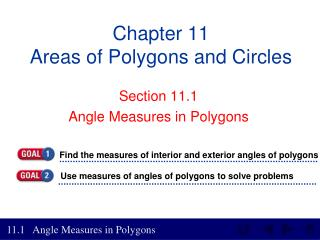DownloadDownload PresentationChapter 11 Areas of Polygons and Circles

# Chapter 11 Areas of Polygons and Circles

Download Presentation## Chapter 11 Areas of Polygons and Circles

- - - - - - - - - - - - - - - - - - - - - - - - - - - E N D - - - - - - - - - - - - - - - - - - - - - - - - - - -
##### Presentation Transcript

1. Use measures of angles of polygons to solve problems Find the measures of interior and exterior angles of polygons Chapter 11Areas of Polygons and Circles Section 11.1 Angle Measures in Polygons

2. Lesson 1 Contents Example 1Interior Angles of Regular Polygons Example 2Sides of a Polygon Example 3Interior Angles Example 4Exterior Angles CA Standard 12.0 Students find and use measures of sides and of interior and exterior angles of triangles and polygonsto classify figures and solve problems. (Key)

3. Angles of Convex Polygons

4. Angles of Regular Polygons

5. Find the measures of interior and exterior angles of polygons n = 6 Measure of one angle =

6. Find the measures of interior and exterior angles of polygons Example 1-1a ARCHITECTUREA mall is designed so that five walkways meet at a food court that is in the shape of a regular pentagon. Find the sum of measures of the interior angles of the pentagon. Since a pentagon is a convex polygon, we can use the Angle Sum Theorem.

7. Find the measures of interior and exterior angles of polygons Example 1-1b Interior Angle Sum Theorem Simplify. Answer: The sum of the measures of the angles is 540.

8. Find the measures of interior and exterior angles of polygons Example 1-1c A decorative window is designed to have the shape of a regular octagon. Find the sum of the measures of the interior angles of the octagon. Answer: 1080

9. Find the measures of interior and exterior angles of polygons Example 1-2a The measure of an interior angle of a regular polygon is 135. Find the number of sides in the polygon. Use the corollary to theorem 11.1 to write an equation to solve for n, the number of sides. Corollary to Theorem 11.1 Multiply by n Distributive Property Subtract 180n from both sides. Divide each side by -45. Answer: The polygon has 8 sides.

10. Find the measures of interior and exterior angles of polygons Example 1-2b The measure of an interior angle of a regular polygon is 144. Find the number of sides in the polygon. Answer: The polygon has 10 sides.

11. Find the measures of interior and exterior angles of polygons Since the sum of the measures of the interior angles is Write an equation to express the sum of the measures of the interior angles of the polygon. Example 1-3a Find the measure of each interior angle.

12. Find the measures of interior and exterior angles of polygons Example 1-3b Sum of measures of angles Substitution Combine like terms. Subtract 8 from each side. Divide each side by 32.

13. Find the measures of interior and exterior angles of polygons Answer: Example 1-3c Use the value of x to find the measure of each angle.

14. Find the measures of interior and exterior angles of polygons Answer: Example 1-3d Find the measure of each interior angle.

15. Find the measures of interior and exterior angles of polygons The Sum is always 360

16. Find the measures of interior and exterior angles of polygons Example 1-4a Find the measures of an exterior angle and an interior angle of convex regular nonagon ABCDEFGHJ. At each vertex, extend a side to form one exterior angle.

17. Find the measures of interior and exterior angles of polygons Example 1-4b The sum of the measures of the exterior angles is 360. A convex regular nonagon has 9 congruent exterior angles. Divide each side by 9. Answer: The measure of each exterior angle is 40. Since each exterior angle and its corresponding interior angle form a linear pair, the measure of the interior angle is 180 – 40 or 140.

18. Find the measures of interior and exterior angles of polygons Example 1-4c Find the measures of an exterior angle and an interior angle of convex regular hexagon ABCDEF. Answer: 60; 120

19. HW # 47Pg 665-668 6-43, 49-61, 63-73 Odd